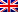# Calinski-Harabasz, Davies-Bouldin, Dunn and Silhouette

Contenus

## Calinski-Harabasz, Davies-Bouldin, Dunn and Silhouette

Calinski-Harabasz, Davies-Bouldin, Dunn, and Silhouette work well in a wide range of situations.

## Calinski-Harabasz index

Performance based on HSE average intra and inter-cluster (Tr):

where B_k is the matrix of dispersion between clusters and W_k is the intra-cluster scatter matrix defined by:

with N the number of points in our data, C_q the set of points of the cluster q, c_q the center of the cluster q, c the center of E, n_q the number of points of the cluster q.

## Davies-Bouldin index

This index treats each cluster individually and seeks to measure how similar it is to the cluster closest to it. The DB index is formulated as follows:

I (c_i) represents the average of the distances between the objects belonging to the cluster C_i and its center. And I (c_i, c_j) represents the distance between the centers of the two clusters C_i and C_j.

For each cluster i of the partition, we look for the cluster j which maximizes the index described as follows:

The best partition is therefore the one that minimizes the average of the value calculated for each cluster. In other words, the best partition is the one that minimizes the similarity between the clusters.

## Dunn's index

Another measure of internal cluster validation that can be calculated as follows is Dunn's Index:

1. For each cluster, calculate the distance between each of the objects of the cluster and the objects of the other clusters
2. Use the minimum of this distance per pair as inter-cluster separation (min.separation)
3. For each cluster, calculate the distance between objects in the same cluster.
4. Use the maximum intra-cluster distance (i.e. maximum diameter) as intra-cluster compactness
5. Calculate Dunn's index (D) as follows:

## Silhouette

Validates performance based on intra and inter-cluster distances:

with a (i) the average dissimilarity with the other data of the cluster and b (i) the weakest dissimilarity with any non-member cluster for each x_i and center of the cluster y:

The silhouette coefficient varies between -1 (worst ranking) and 1 (best ranking). Silhouette's overall average is often calculated.FRFRENES